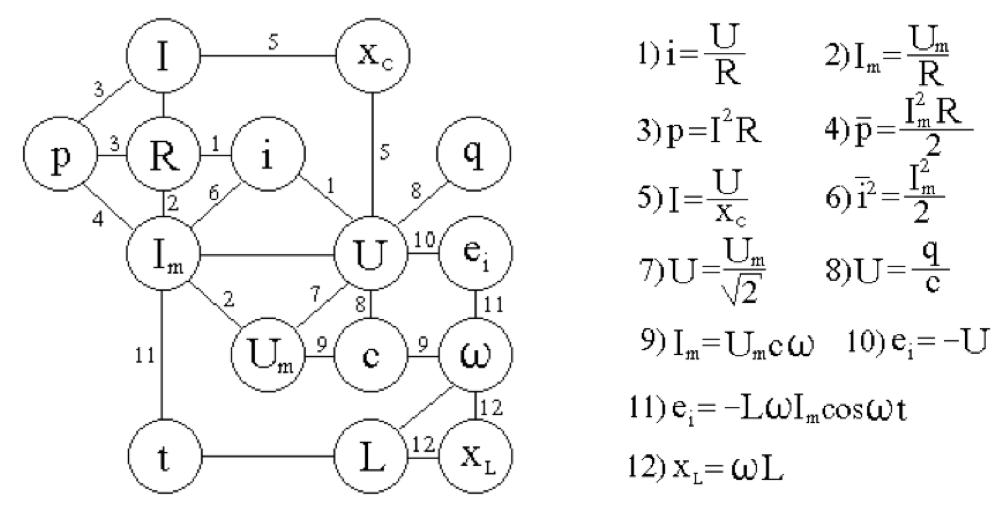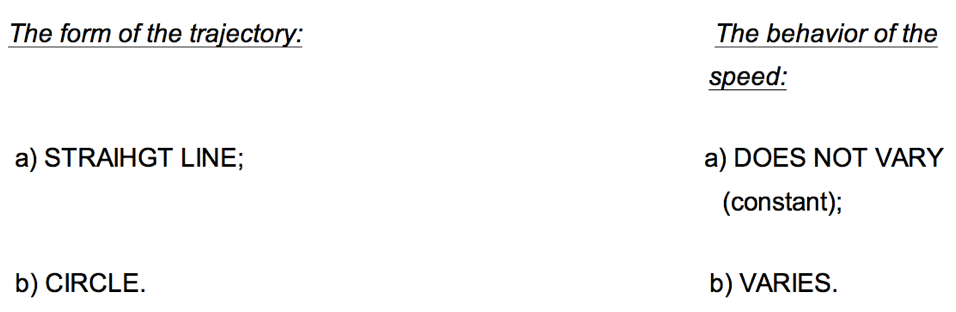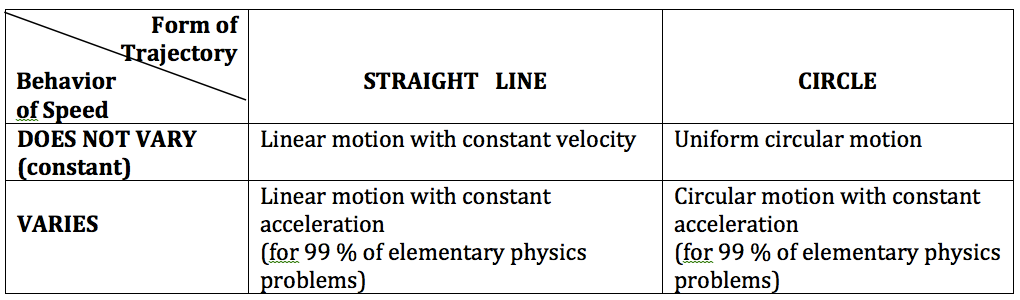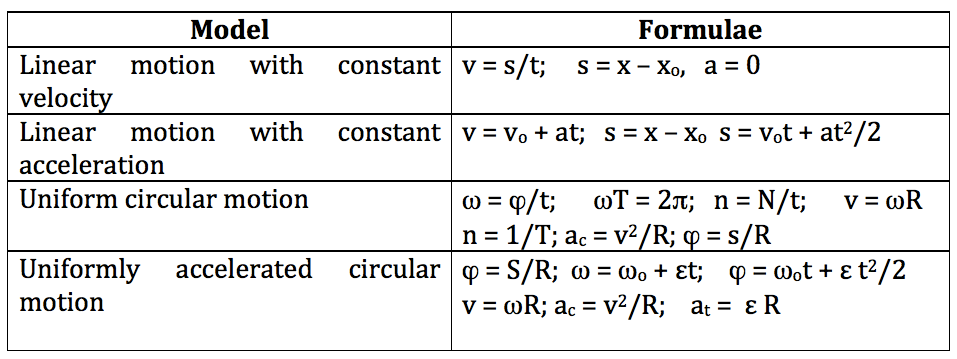<<back to publications<< …...................>> to Teach0logy.xyz>>

Learning aides for students taking physics.

By Valentin Voroshilov

Recently I have describe a map of operationally connected categories (MOCC) as an instrument for classifying physics problems,

In my teaching experience, MOCCs also proved to be a helpful teaching instrument.

One of the use of MOCCs is having it drawn for a problem being solved in front of a class. The visualisation of logical connections between quantities helps students to grasp the logic of creating of the solution. Some students develop a habit of using MOCC when study physics.

Below you see an example of a MOCC developed by a student.The pedagogical use of MOCCs is more efficient if combined with the use of other learning aids.

(I) A physicist always compares a given problem against a set of previously known situations (models), and to do that he or she uses a certain classification (usually on a subconscious level). A teacher can show students this kind of mental work as a part of the problem solving process.

An example of a model classification for problem solving

Any process of recognition is always based on some classification parameters and their values.

For example, in elementary Kinematics, to identify the model we deal with two main parameters of classification: 1. The form of the trajectory; 2. The behavior of the speed. Within the standard framework, the following values of the parameters are important:In relation to the values of the parameters, four main kinematics models can be identified.We cannot use the table to solve any problem on Kinematics, but we can use the principle!

(II) When the model is identified; then we can assemble a set of the most important physical quantities needed to investigate the model, for example as shown in the table below (these are the physical quantities which are most probably involved in solving a problem on Kinematics).(III) Finally, we can write equations which (most probably) will be useful for solving a problem. For example, the table below represents the correspondence between the kinematical models and the formulae which can be used for them.

It is important to emphasize that this step – choosing equations – is usually the last step of the analysis of the problem (when done by an expert). After this step physics is done, merely mathematical calculations are left.(IV) When a physicist reads a problem he/she translates it immediately (and intuitively) into the text written using a general language. For example, a problem “For a takeoff a plain needs to reach speed of 100 m/s. The engines provide acceleration of 8.33 m/s2. Find the time it takes for the plain to reach the speed.” will be translated as: “A body moves from rest with a constant acceleration (which is given) and at some instant of time (which is unknown) has a specific speed (which is given).”

A special tool – “a dictionary” – will help students to conduct this kind of translation.

An example of  “a dictionary”:All the learning aids (a.c.a. teaching tools) describe above can be used as a part of the tool for measuring learning outcomes of students taking physics course: students can be offered identify a model, translate a problem, draw a MOCC for the given problem, to follow a general algorithm for solving physics problems.

You also may find useful my description of a general thinking process  which is a part of a reasoning every expert problem solver uses when solving a physics problem (at http://teachology.xyz/sp.htm).

Literature

Voroshilov V., “Universal Algorithm for Solving School Problems in Physics” // in the  book "Problems in Applied Mathematics and Mechanics". - Perm, Russia, 1998. - p. 57.

Voroshilov V., “Application of Operationally-Interconnect Categories for Diagnosing the Level of Students' Understanding of Physics” // in the book “Artificial Intelligence in Education”, part 1. - Kazan, Russia, 1996. - p. 56.

Voroshilov V., “Quantitative Measures of the Learning Difficulty of Physics Problems” // in the book “Problems of Education, Scientific and Technical Development and Economy of Ural Region”. - Berezniki, Russia, 1996. - p. 85.

<<back to publications<< …................... >> to Teach0logy.xyz>>